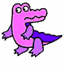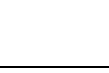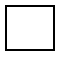Skill 1E
Number Sentence Understanding

A number sentence is the textbook term given to a numerical math problem such as 2+2=4.
It is a "sentence" that only uses numbers and operation signs such as plus or minus and an equals sign. Here, we want to make sure your student can look at two groups of objects and make a number sentence from the objects.  The number sentence is what allows a student to figure out the answer to every word problem.  The words "number sentence" must be understood without hesitation.

Below are two groups of alligators.
Write a number sentence that would go with the picture.